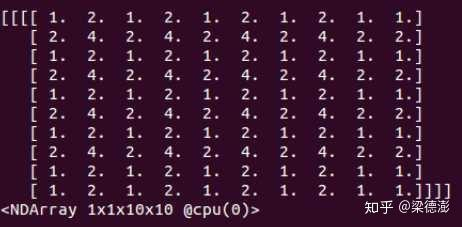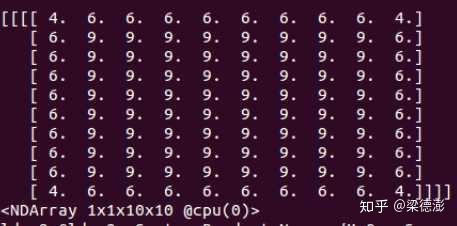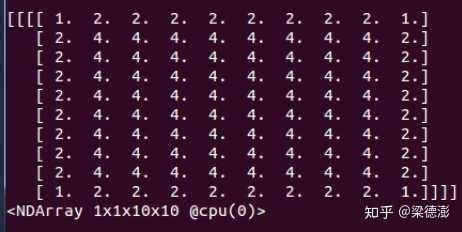# 深入理解深度学习中的反(转置)卷积

https://arxiv.org/pdf/1603.07285.pdf

【超分辨率】Efficient Sub-Pixel Convolutional Neural Network

## 卷积前后向传播实现细节¶

Caffe :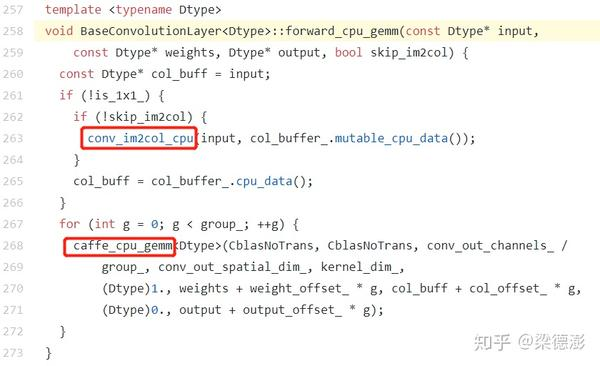MXNet :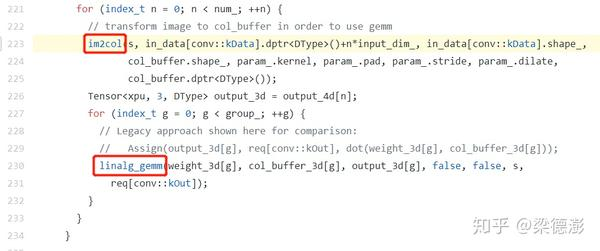im2col 实现细节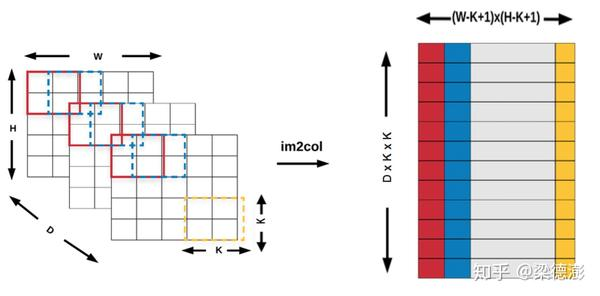过程如入上图所示，窗口从左到右上到下的顺序在每个输入通道同步滑动，每个窗口内容按行展开成一列，然后再按通道顺序接上填到buffer对应的列，且buffer 按从左到右顺序填写。

Hout = $H + 2p - K$/s + 1 = H-K+1

Wout &= $W + 2p - K$/s + 1 = W-K+1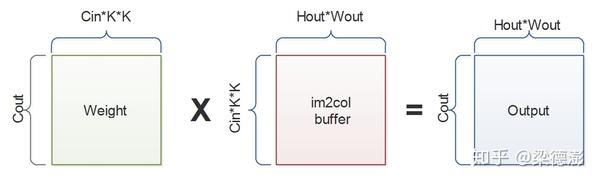之前先要先根据pad在输入边缘补一圈0，然后再根据步长s去取每个卷积的位置填入buffer里面。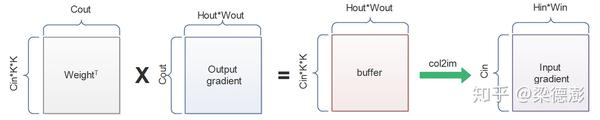## 反卷积的两种实现方式¶

### GEMM + col2im¶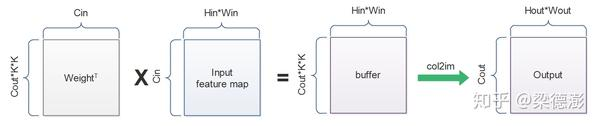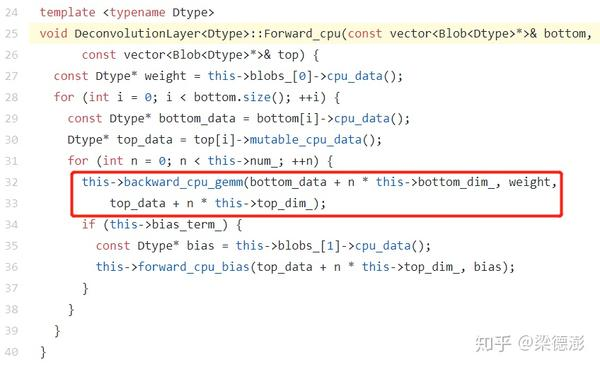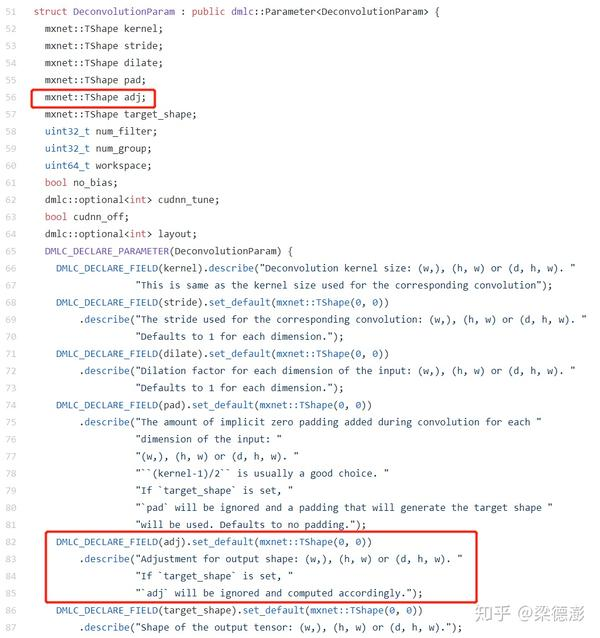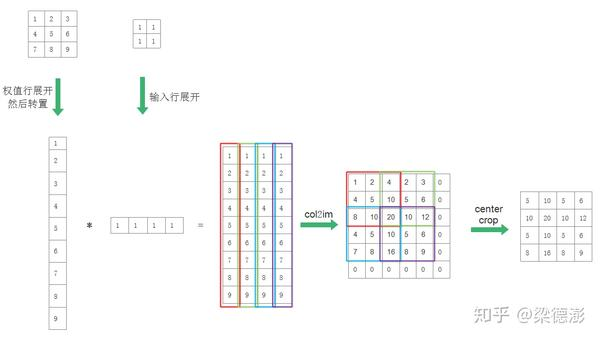import mxnet as mx
import numpy as np

data_shape = (1, 1, 2, 2)
data = mx.nd.ones(data_shape)

deconv_weight_shape = (1, 1, 3, 3)
deconv_weight = mx.nd.ones(deconv_weight_shape)
deconv_weight[:] = np.array([1,2,3,4,5,6,7,8,9]).reshape((1,1,3,3))

# deconvolution forward
data_deconv = mx.nd.Deconvolution(data=data, weight=deconv_weight,
kernel=(3, 3),
stride=(2, 2),
num_filter=1)
print(data_deconv)

# convolution backward
data_sym = mx.sym.Variable('data')
conv_sym = mx.sym.Convolution(data=data_sym, kernel=(3, 3), stride=(2, 2), pad=(1, 1), num_filter=1, no_bias=True, name='conv')
executor = conv_sym.simple_bind(data=(1, 1, 4, 4), ctx=mx.cpu())
deconv_weight.copyto(executor.arg_dict['conv_weight'])
executor.backward(mx.nd.ones((1, 1, 2, 2)))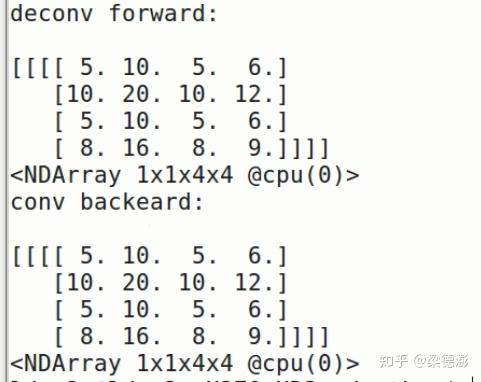### 输入插空补0+卷积¶

https://arxiv.org/pdf/1603.07285.pdf

4.6节给出的推导公式，先从卷积前向方向来看，假设输入是，卷积核大小、步长和pad分别是，则卷积输出大小计算公式如下：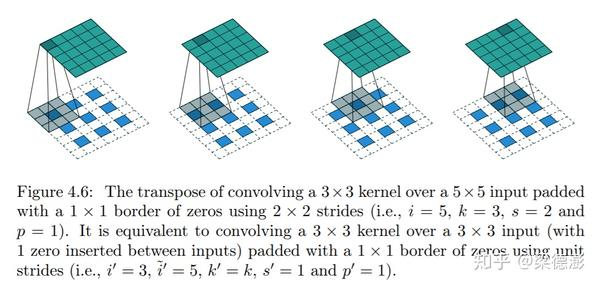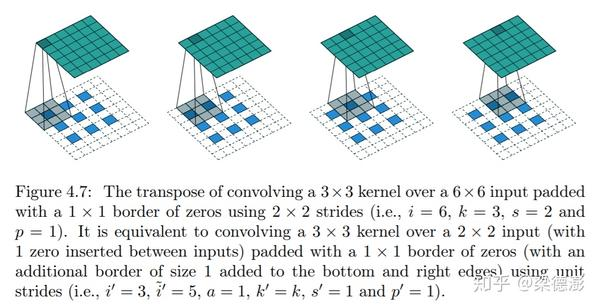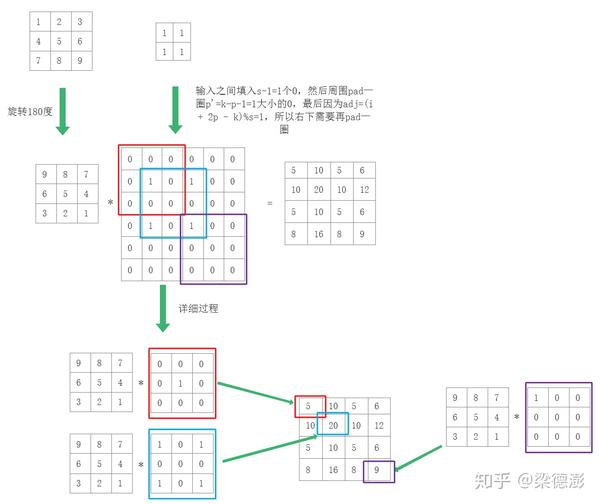https://github.com/alibaba/MNN/blob/master/source/backend/metal/MetalDeconvolution.metal#L83

#define UP_DIV(x, y) (((x) + (y) - (1)) / (y))
#define ROUND_UP(x, y) (((x) + (y) - (1)) / (y) * (y))

kernel void deconv_depthwise(const device ftype4 *in        [[buffer(0)]],
device ftype4 *out             [[buffer(1)]],
constant deconv_constants& cst [[buffer(2)]],
const device ftype4 *wt        [[buffer(3)]],
const device ftype4 *biasTerms [[buffer(4)]],
if ((int)gid.x >= 4 || (int)gid.y >= 4) return;

float4 result = float4(biasTerms[(short)gid.z]);

short oy = (short)gid.y + 1;
// 第一个输出:1，第6个输出：2
short ox = (short)gid.x + 1;
// 第一个输出:1，第6个输出：2
short max_sy = min((2 - 1) * 2, oy / 2 * 2);
// 第一个输出:0，第6个输出：2
short max_sx = min((2 - 1) * 2, ox / 2 * 2);
// 第一个输出:0，第6个输出：2
short min_ky = UP_DIV(oy - max_sy, 1);
// 第一个输出:1，第6个输出：0
short min_kx = UP_DIV(ox - max_sx, 1);
// 第一个输出:1，第6个输出：0

if ((oy - min_ky * 1) % 2 == 0 && (ox - min_kx * 1) % 2 == 0) {
short min_sy = max(0, ROUND_UP(oy + 1 - 3 * 1, 2));
// 第一个输出:0，第6个输出：0
short min_sx = max(0, ROUND_UP(ox + 1 - 3 * 1, 2));
// 第一个输出:0，第6个输出：0
short max_ky = (oy - min_sy) / 1;
// 第一个输出:1，第6个输出：2
short max_kx = (ox - min_sx) / 1;
// 第一个输出:1，第6个输出：2
short min_iy = (oy - max_ky * 1) / 2;
// 第一个输出:0，第6个输出：0
short min_ix = (ox - max_kx * 1) / 2;
// 第一个输出:0，第6个输出：0

for (auto ky = max_ky, iy = min_iy; ky >= min_ky; ky -= 2, iy += 2) {
for (auto kx = max_kx, ix = min_ix; kx >= min_kx; kx -= 2, ix += 2) {
auto wt4 = wt[ky * 3 + kx];
auto in4 = in[iy * 2 + ix];
result += float4(in4 * wt4);
}
}
}
}


Tensor中数据摆放顺序NC4HW4是什么意思，只知道NCHW格式，能解释以下NC4HW4格式吗？

## 反卷积的缺点¶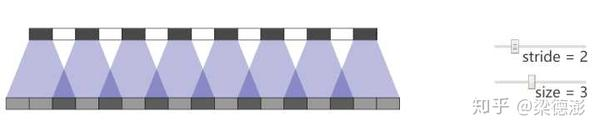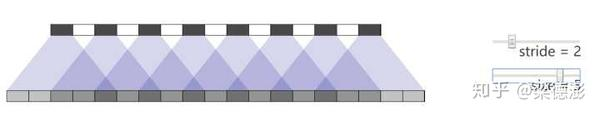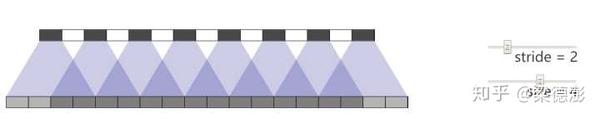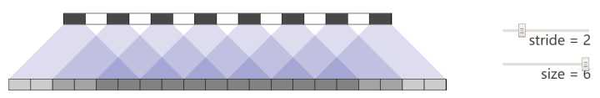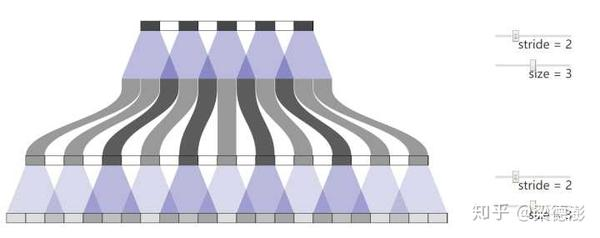import mxnet as mx

batch_size = 1
in_channel = 1
height = 5
width = 5

data_shape = (batch_size, in_channel, height, width)
data = mx.nd.ones(data_shape)

out_channel = 1
kernel_size = 3
deconv_weight_shape = (in_channel, out_channel, kernel_size, kernel_size)
deconv_weight = mx.nd.ones(deconv_weight_shape)

stride = 2
up_scale = 2
data_deconv = mx.nd.Deconvolution(data=data, weight=deconv_weight,
target_shape=(height * up_scale, width * up_scale),
kernel=(kernel_size, kernel_size),
stride=(stride, stride),
num_filter=out_channel)
print(data_deconv)

data_upsample = mx.nd.contrib.BilinearResize2D(data=data, scale_height=up_scale, scale_width=up_scale)
conv_weight_shape = (out_channel, in_channel, kernel_size, kernel_size)
conv_weight = mx.nd.ones(conv_weight_shape)
pad = (kernel_size - 1) / 2
data_conv = mx.nd.Convolution(data=data_upsample, weight=conv_weight,
kernel=(kernel_size, kernel_size),
print(data_conv)